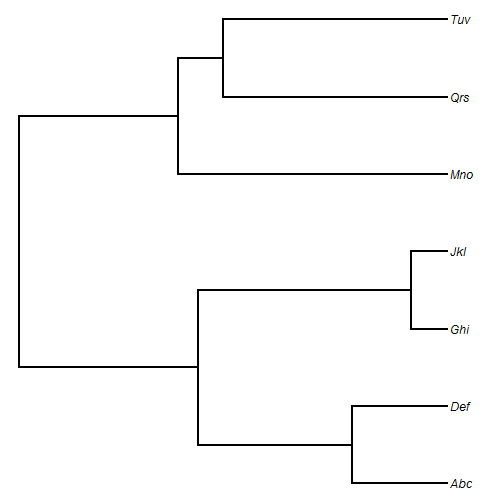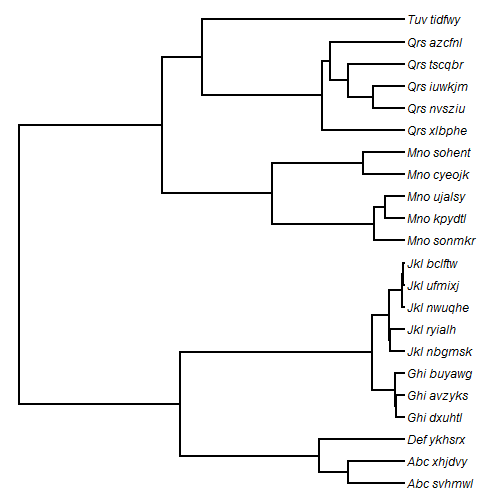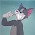## Tuesday, October 27, 2015

### Simulating a species tree from a genus tree in which the genera arise (stochastically) under a Yule process

Today, Karla Shikev asked the following question:

I need to add species go a genus-level phylogeny, such that the generated nodes are consistent with a Yule process. I tried phytools' add.species.to.genus function, but the generated branch lengths tend to be too recent.

`add.species.to.genus` adds tips randomly - not under some model. However, if we have a purely genus-level tree (that is, a tree in which only genera are represented) and we want to produce random species trees, in which each subtree arose by a Yule process - then this is not too hard either.

Here is a quick demo:

``````library(phytools)
## first let's simulate our genus tree:
genus.tree<-pbtree(n=7,tip.label=c("Abc","Def","Ghi","Jkl","Mno","Qrs","Tuv"))
## here it is:
plotTree(genus.tree,ftype="i")
``````Next, let's simulate some random species within each genus to attach to our tree. Here I simulate 1-5 species per genus; however, note that if there is only one species in a genus, then the tip of our genus tree will simply be renamed.

``````tips<-c()
for(i in 1:Ntip(genus.tree)){
n.genus<-sample(1:5,1)
for(j in 1:n.genus) tips<-c(tips,paste(genus.tree\$tip.label[i],paste(sample(letters,6),collapse=""),sep="_"))
}
## these are the tips we want for our species tree:
tips
``````
``````##   "Abc_svhmwl" "Abc_xhjdvy" "Def_ykhsrx" "Ghi_dxuhtl" "Ghi_avzyks"
##   "Ghi_buyawg" "Jkl_nbgmsk" "Jkl_ryialh" "Jkl_nwuqhe" "Jkl_ufmixj"
##  "Jkl_bclftw" "Mno_sonmkr" "Mno_kpydtl" "Mno_ujalsy" "Mno_cyeojk"
##  "Mno_sohent" "Qrs_xlbphe" "Qrs_nvsziu" "Qrs_iuwkjm" "Qrs_tscqbr"
##  "Qrs_azcfnl" "Tuv_tidfwy"
``````

OK, now let's imagine how we want to create our species tree in which each genus arose via a Yule process. We can simply take the genus tree and glue on a Yule tree somewhere along the terminal edge leading to each tip in the genus tree! Here is an example function in which this is done at a random position along that edge - but this needn't be the case, and could be modified easily:

``````genus.to.pbSpeciesTree<-function(tree,tips){
N<-Ntip(tree)
genera<-tree\$tip.label
for(i in 1:N){
jj<-grep(paste(genera[i],"_",sep=""),tips)
nn<-which(tree\$tip.label==genera[i])
if(length(jj)>1){
h<-runif(n=1)*tree\$edge.length[which(tree\$edge[,2]==nn)]
tree\$edge.length[which(tree\$edge[,2]==nn)]<-
tree\$edge.length[which(tree\$edge[,2]==nn)]-h
sub.tree<-pbtree(n=length(jj),scale=h,tip.label=tips[jj])
tree<-bind.tree(tree,sub.tree,where=nn)
} else tree\$tip.label[nn]<-tips[jj]
}
tree
}
species.tree<-genus.to.pbSpeciesTree(genus.tree,tips)
plotTree(species.tree,ftype="i")
``````As an alternative to rescaling, we could have simulated conditioned on time & a birth rate; however I'm not sure it would make much difference, and it would require that some birthrate be supplied.

That's it!

1.2.